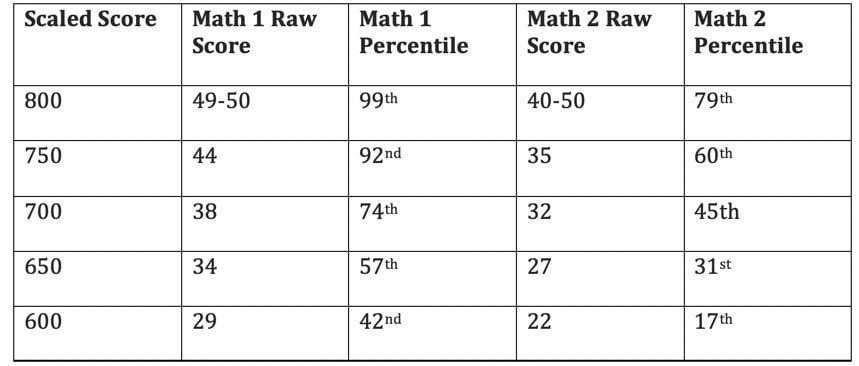April 16, 2019, by

# As we near the end of the school year, a lot of high schoolers are sweating over the question, “Should I take the Math 1 or Math 2 Subject Test?”

Both College Board tests are 60 minutes long; both are multiple choice, and both allow calculators.  Both tests also have 50 questions that go in order of increasing difficulty, and they are scored on the standard 800 scale just like the individual SAT sections.

However, the tests differ in terms of content assessed.  The Mathematics Level 1 Subject Test covers material commonly learned in Geometry, Algebra 1 and Algebra 2, whereas the Mathematics Level 2 Subject Test includes Pre-calculus.  The best way to know whether or not you should attempt the Math 2 over the Math 1 test is to take a timed practice Math 2 test released by the College Board.  (Non College Board tests are not as reliable.)

In addition to the content covered, there are a few things to think about that may help steer you in one direction or another. Above all, the Math 2 test is significantly harder than the Math 1 test. The Math 2 manages to be trickier by testing more specific material (formulas, equations, problem solving methods), and by being much harder to finish in the 60-minute time limit. Traditionally, Math 2 has been a test for top math students – for future physics, engineering or math majors or those who want to show that they excel in math. This doesn’t mean you have to be the best in your class to attempt the Math 2, but the test is not for everyone.

## Other factors to consider

Another factor to consider is the scoring scale. Curiously, the Math 1 has a more forgiving percentile scale, while the Math 2 has a more forgiving score scale.

For example, to get an 800 on the Math 1 test, you can only miss one question, but you would be in the 99th percentile (meaning you scored better than 99% of students taking the test across the country). On the Math 2 test, you can typically skip 3-5 questions and still score an 800, yet you would only be in the 79th percentile. The tougher percentile scale on the Math 2 test is a reflection of the fact that the students taking the test are very strong in math. This means that roughly 21% of students taking the Math 2 test score an 800.

Here is a table illustrating some scoring and percentage examples. On the subject tests, there is a wrong answer penalty, so the raw score is calculated by taking the number of questions correct and subtracting one quarter of a point for each wrong answer.  Omitted questions neither gain nor lose a point.  The following table shows some of the differences in the scoring:## Bottom Line

• If you’re applying to MIT, Harvey Mudd, CIT or an engineering college, you will probably need scores from the Math 2 test.
• If you don’t think you will be able to score over a 700 on the Math 2, consider taking the Math 1.  You might achieve a similar score, but a much higher percentile.
• The Math 1 and Math 2 tests are offered on seven test dates throughout the school year, from August to June.  If you’re in the 10th grade and not quite ready now, consider taking either test next year.
• Students who take the Math 2 after taking AP AB or BC Calculus often find that they have forgotten some Pre-Calculus.  However, students who take it after a month of Calculus (in October or November), often do well (if they are doing well in class).

Again, if you still have questions about which test is best for you, schedule a free proctored practice test at Marks Education, and then consult your tutor.  Good luck!!